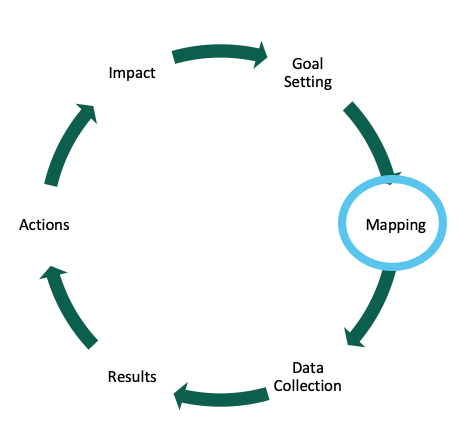# Academic Assessment Cycle - Curriculum MappingThe second stage in the continuous improvement and assessment process is mapping. Curriculum mapping requires developing an intentional program of study for the students which allows them to meet the department’s standards for each Student Learning Outcome.

Mapping curriculum in an intentional way allows departments and faculty to plan where each Student Learning Outcome is introduced, reinforced, and mastered.

Each Student Learning Outcome should be introduced, reinforced, and mastered throughout the required core courses of the program’s curriculum.

It is useful to visualize curriculum maps. A simple visualization can be created using Microsoft Word or Excel by making a table where the Student Learning Outcome represents a column and courses required in the program represent rows. The first step is to indicate, with an X, the courses where each Student Learning Outcome is covered.

 Student Learning Outcome (SLO) 1 Student Learning Outcome (SLO) 2 Student Learning Outcome (SLO) 3 Course A X X Course B X X X Course C X X Course D X X

Then review the table and indicate the degree to which each Student Learning Outcome is addressed in each course. I can be used for introduced, R for reinforced, and M for mastered.

 Student Learning Outcome (SLO) 1 Student Learning Outcome (SLO) 2 Student Learning Outcome (SLO) 3 Course A I I Course B R I R Course C R M Course D M M

Colors can also be utilized to represent how the curriculum map flows and make it more aesthetically pleasing.

 Student Learning Outcome (SLO) 1 Student Learning Outcome (SLO) 2 Student Learning Outcome (SLO) 3 Course A I I Course B R I R Course C R M Course D M M

For each Student Learning Outcome, a course should introduce the concept before it is expected the students will have it reinforced or mastered within a curriculum.

If a Student Learning Outcome is not mastered within a curriculum there may be one of two issues: 1) the Student Learning Outcome may not be valuable to the program, or 2) the program may need to be restructured.

Ideally, summative assessments will take place in courses where a Student Learning Outcome is mastered.

For further reading on Course Mapping please visit the National Institute for Learning Outcomes Assessment